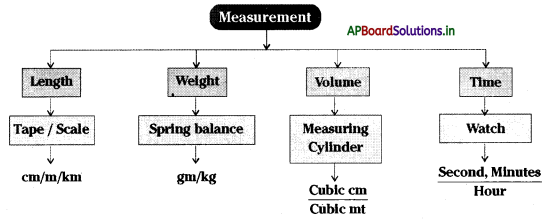# AP Board 6th Class Science Notes Chapter 7 Let us Measure

Students can go through AP State Board 6th Class Science Notes Chapter 7 Let us Measure to understand and remember the concept easily.

## AP State Board Syllabus 6th Class Science Notes Chapter 7 Let us Measure

→ Foot space, hand space, cubit are conventional methods to measure the lengths.

→ The standard unit of length is Meter.

→ The smallest unit of length is centimeter/millimeter (cm/mm) and the larger unit of length is Meter / Kilometer (m/km).

→ The measurement of the extent of plane surface occupied by an object is Area.

→ A square centimeter (cm2) is a standard unit to measure the area of a surface.

→ Volume is a measurement of the extent of space occupied by an object.

→ The volume of solids is measured in cubic meters, cubic centimeters.

→ The volume of liquids is measured in liters or milliliters.

→ We need standard instruments to measure lengths accurately.→ The area is a measure of the extent of the plane surface occupied by an object.

→ France made a certain length of rod made of special material of platinum-iridium called a meter.

→ The original scale is preserved in the museum of France.

→ In our daily life, we use different types of measurements like plain type roll type centimeter scale of different sizes made up of wood iron, and plastic.

→ While measuring the length of objects the zero point of scale should be consisting the starting of an object

→ The perimeter of the bucket and perimeters of Kadai is examples of curved surfaces

→ For measuring the volume of liquids in laboratories like milk and oil etc., they use measuring cylinders

→ The volume of the box is = Length × Breadth × Height

→ Measure:‘ The unit used to know the size and weight of an object

→ The standard unit of area: A level of quality

→ Volume: The extent of space occupied by a body→ Regular Surface: A systematic area whose length and breadth can easily measure

→ Irregular Surface: An unsystematic, changeable area whose length and breadth cannot easily measure

→ Rectangular body: A plane figure with four straight sides and four right angles. with opposite sides are parallel and of equal length.

→ Measuring cylinder: A long hollow tube which is using to measure the volume of liquids and solids

→ Graph Paper: A paper with equal designs and used to measure the area of irregular surface bodies

→ Yard: The distance between nose and tip of the middle fingers

→ Area: The surface occupied by an object

→ Handspan: The gap between thumb line and little finger

→ Cubit: The length between elbow and tip of the middle finger

→ Tarragona: A famous rural game in ÆP. gilildanda (karrabilla)

→ Feet: 12 inches of length in feet→ Scale: The universal unit to measure length and width

→ Meter: 100 cm length is a meter→ “Live and Let Live”:
You must have noticed that the volumes of liquids are written ¡ri ml while those of solids are written in cm3. Do you know the relation between these two units? The two units are related as follows: 1 ml = 1 cm3